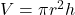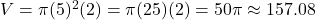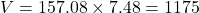Question

6. A kid’s pool is cylindrical and has a radius of 5 feet and a height of 2 feet. If a cubic foot holds about 7.48 gallons of water, how many gallons of water does the kid’s pool hold?

1.huyenthanh

1,175,108gallons of water

Step-by-step explanation:

do the volume of the cylindrical pool that is base area multiply by height to get 157.1 feet then you do 1cubic feet is 7.48 gallons what about 157.1 feet cubic

2.RuslanHeatt

1175 gallons (rounded)

Step-by-step explanation:

The volume of a cylinder is given by the formula.

r is the radius, h is the height.cubic feet

Each cubic foot contains 7.48 gallons, sogallons (rounded).

Just for fun, each cubic foot weighs 62.4 pounds, so the water in the pool weighs about 9801 pounds — more than 4 tons!